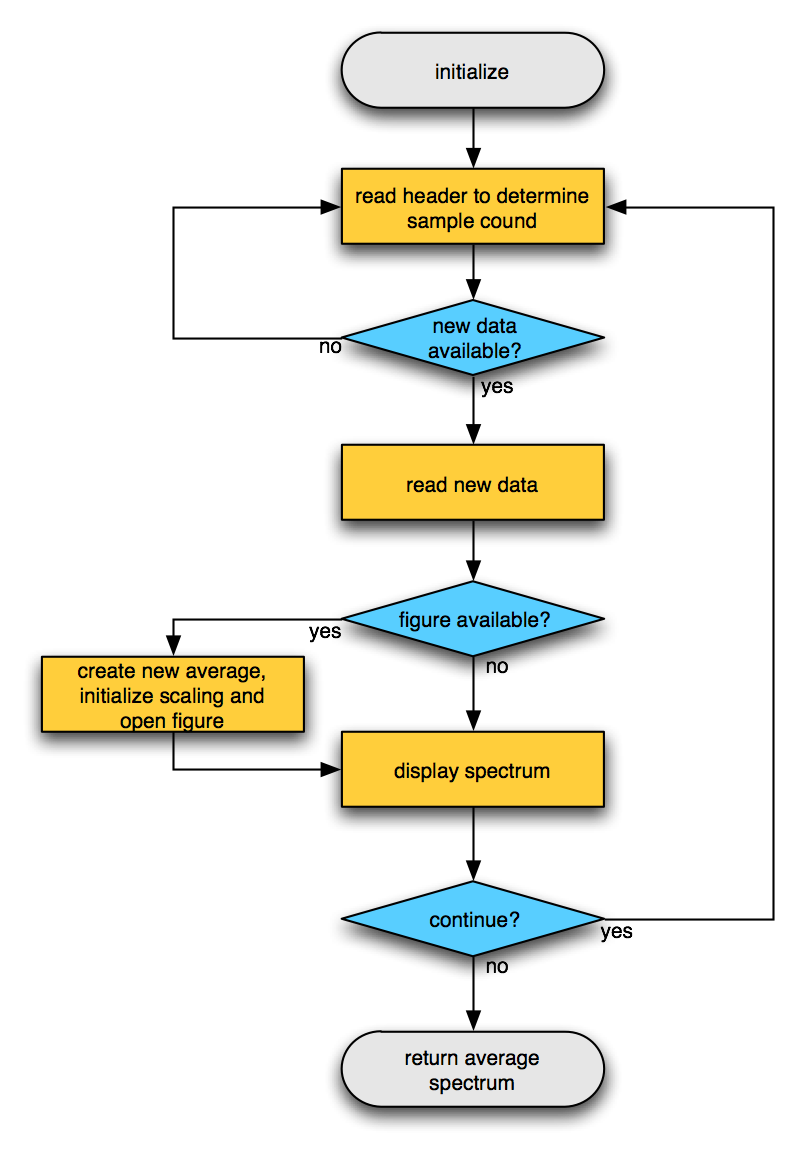Tags: example realtime

# Example real-time power estimate

The ft_realtime_powerestimate function reads data in small chunks and performs a spectral estimation for each chunck. The output of this function is a constantly updating figure with the power spectrum, averaged over the selected channels.

## Flowchart## Example use

The easiest way to try out the ft_realtime_powerestimate example is by starting two MATLAB sessions. In the first session you create some random signal and write it to the buffer by means of ft_realtime_signalproxy:

``````cfg                = [];
cfg.channel        = 1:10;                         % list with channel "names"
cfg.blocksize      = 1;                            % seconds
cfg.fsample        = 250;                          % sampling frequency, Hz
cfg.lpfilter       = 'yes';                        % apply a low-pass filter
cfg.lpfreq         = 20;                           % filter frequency, Hz
cfg.target.dataset = 'buffer://localhost:1972';    % where to write the data
ft_realtime_signalproxy(cfg)
``````

In the second MATLAB session you start the ft_realtime_powerestimate and point it to the buffer:

``````cfg                = [];
cfg.blocksize      = 1;                            % seconds
cfg.foilim         = [0 30];                       % frequency-of-interest limits, Hz
cfg.dataset        = 'buffer://localhost:1972';    % where to read the data from
ft_realtime_powerestimate(cfg)
``````

After starting the ft_realtime_powerestimate, you should see a figure that updates itself every second. That figure contains the powerspectrum of the simulated random number signal. If you close the figure, the figure will re-appear and start all over again with the automatic scaling of the vertical axis.

You can also start the two MATLAB sessions on two different computers, where on the second you would then point the reading function to the first computer.

## MATLAB code

The code for ft_realtime_powerestimate is included in the FieldTrip release under `fieldtrip/realtime/example` and can also be found on GitHub.